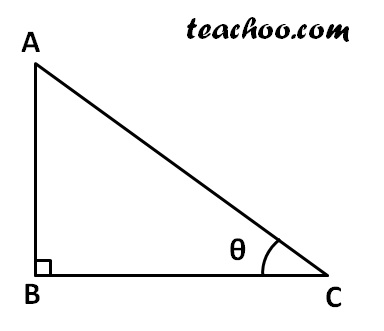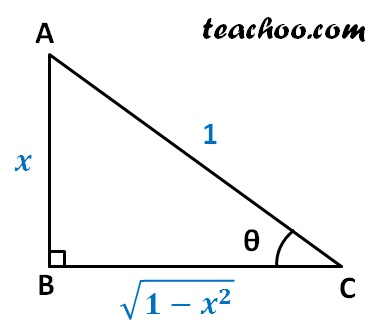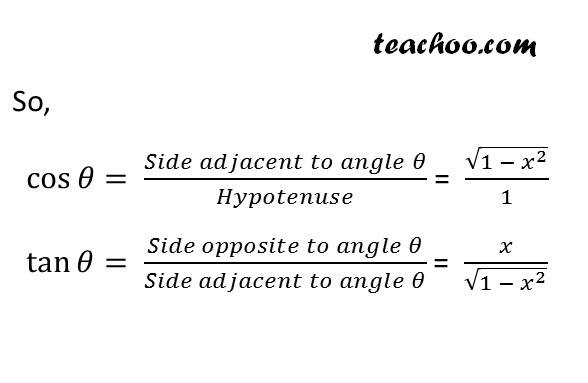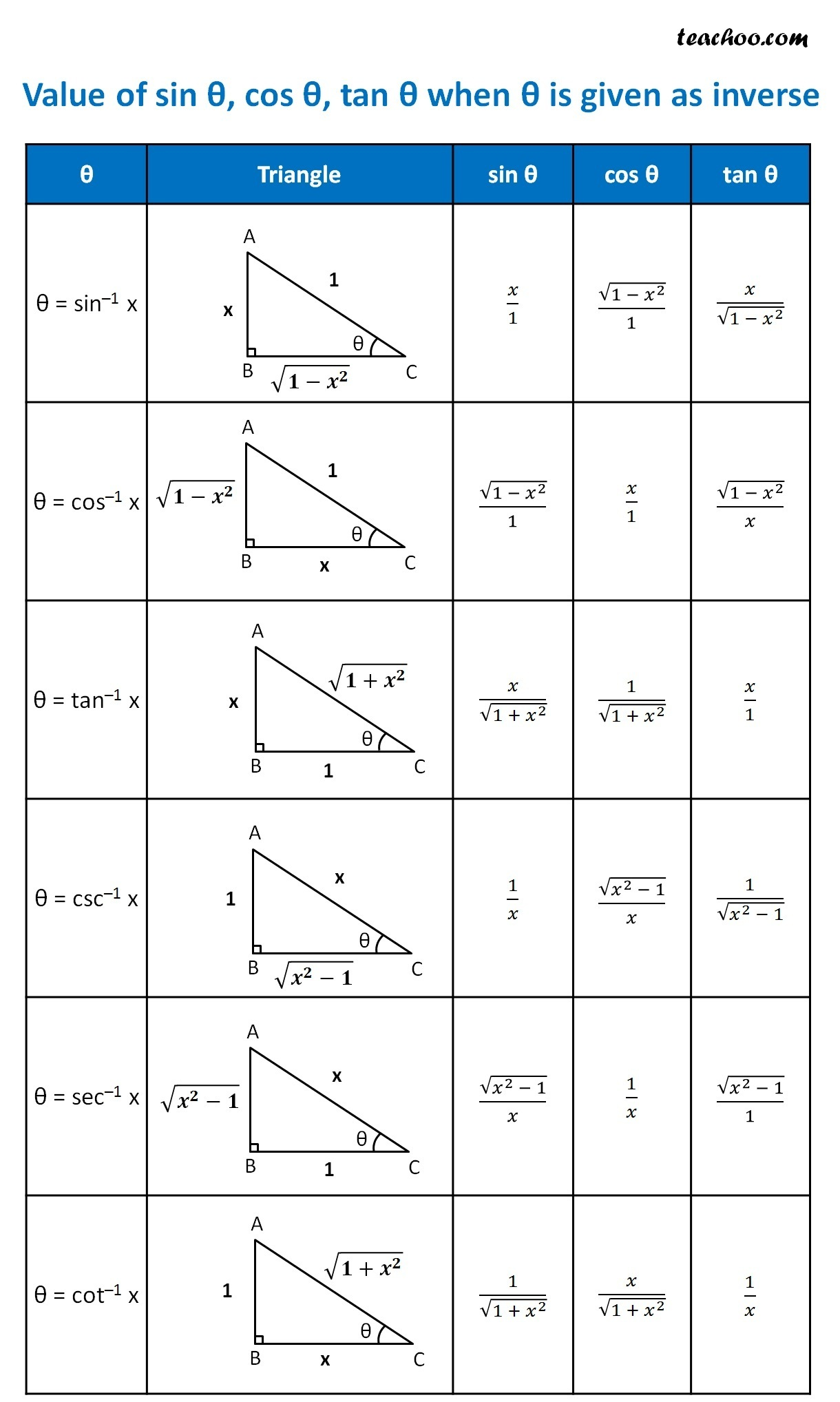Definition

Chapter 2 Class 12 Inverse Trigonometric Functions
Concept wise

Suppose we are given

θ = sin –1 xWe need to find sin θ , cos θ and tan θ

Now,

θ = sin –1 x

So,

sin θ = x

sin θ = x/1

And we know that

sin θ =  (Side opposite to angle θ)/Hypotenuse

x/1 = AB/AC

So, AB = x, AC = 1

Now, in right triangle Δ ABC

By Pythagoras Theorem

AC 2 = AB 2 + BC 2

1 2 = x 2 + BC 2

1 – x 2 = BC 2

BC = √(1-x 2 )## Value of sin θ , cos θ , tan θ when θ is given as inverseGet live Maths 1-on-1 Classs - Class 6 to 12